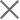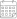Subscribe

### Indexes

Quarter 3-4, 2020 | Energy Market Review22 June 2021

In the third and the fourth quarters of 2020, Georgian power plants generated 2,958 mln. and 2,594 mln. kWh of electricity, respectively. This represents a 9.6% decrease and 2.4% increase in total generation compared to the corresponding periods of the previous year (in 2019, the total generation in Q3 was 3,273 mln. kWh and 2,534 mln. kWh in Q4). On an annual basis, the reduction in generation in the third quarter of 2020 was derived from a 7.6%, 9.6%, and 5.4% decline in thermal, hydro, and wind power generation, respectively. The increase in production in the fourth quarter is related to the 14.1% and 0.07% increase in thermal and wind power generation, more than offsetting the 3.4% decrease in hydropower generation.

In the third and fourth quarters of 2020, electricity consumption on the local market amounted to 2,824 mln. (Q3) and 2,916 mln. kWh (Q4) (-13.8% and -2.8% compared to the third and fourth quarters of 2019, respectively) (Figure 1). Total power generation exceeded consumption by 134 mln. in the third quarter of the year and lagged by 322 mln. kWh in the fourth quarter. In percentage terms, in Q3 there was a generation surplus amounting to 4.5% of total generation, while in Q4 there was a generation deficit of 12.4% of total generation. In 2019 the difference between total generation and consumption resulted in a shortage in the third quarter of 6 mln. kWh, around 0.2% of the total generation, and in the fourth quarter shortage of 466 mln. kWh, around 18.4% of the total generation.